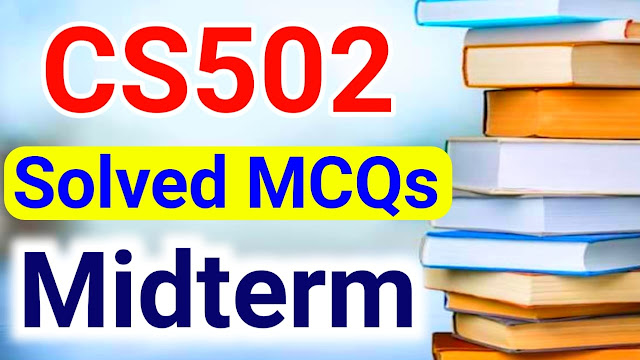# CS502 Solved MCQs With Answers For Midterm

In this post, students will read CS502 Solved MCQs for midterm so students please read and complete this post and also try to share with other class fellows students who also have this CS502 book. Students we have uploaded many books that solved MCQs so please also check them by visiting our entire website. This is the CS502 - Fundamentals of Algorithms Solved MCQs for CS502 book students who join this Virtual University and have the CS502 book. Many students feel that VU study is very difficult because Virtual Univerity is a distance-based university as well as an online education system but some students face problems don't worry we will help you by providing VU all books study solutions then you will easily prepare for Virtual University Exams. Students if you like these  With Answers then you can share them on WhatsApp groups and Facebook groups. Also, Read CS502 Solved MCQs Midterm.

### CS502 - Fundamentals of Algorithms Solved MCQs

 Post Title CS502 Solved MCQs With Answers For Midterm Book Code CS502 Current/Past Current CS502 Solved MCQs With Answers Mid/Final CS502 Solved MCQs With Answers For Midterm Also, Read CS502 Lecture Wise MCQs Midterm Also, Read CS502 Quiz 1 Solution

## CS502 Solved MCQs With Answers For Midterm

Array divided into …………sub arrays while copying seve technique to selection problem.  (CS502)

3

2

1

4

In ………..we have to find the rank of an element from a given input.  (CS502)

Plane sweep algorithm

Merge sort algorithm

Brute force technique

Selection problem

The process of ……….ends when you are left with such tiny places remaining that it is trivial to solve them.  (CS502)

Divide and conquer

Axis sweep

Plan sweep

Brute force

While analyzing algorithms……………and ………are usually considered difficult to calculate.  (CS502)

Floor,ceiling

Row,column

Finite ,infinite

Graph ,tree

The rank of an element can be defined as ……………(CS502)

One minus the number of elements that are smaller

One plus the number of elements that are smaller

Two plus the number of elements that are greater

Two minus the number of elements that are smaller

The algorithm is a sequence of computational steps that ……….. The input into output.  (CS502)

Merge

Transform

Assign

Integrator

In 2-D maxima problem. A point p is said to be dominated by point q if …………(CS502)

px>qx and p,y>q.y

P.x = and p.y = q.y

Px = q.x and p.y = q.y

bp.x <= q.x and p.y <= q.y

The time assumed for each basic operation to execute on the RAM model of computation is …………..(CS502)

Variable

Constant

Infinite

Continuous

In the analysis of algorithms ………plays an important role.   (CS502)

Money

Time

Growth rate

Text analysis

For small values of n…………algorithm is last enough.  (CS502)

Reap sort

Quicksort

Any

Merge sort

Two functions are said to be asymptotically equivalent if they have ………….(CS502)

Been proved as equivalent

Some number of polynomials

Some input

Some growth rates for large n

The approach of solving geometric problems by sweeping a line across the plane is called ………….sweep.  (CS502)

Line

Plane

Cube

Box

The brute force algorithm for 20 maxima runs in ordered O(..............) time.  (CS502)

n*n

n(logn)

N

N3

…………is not a characteristic of random access machines.  (CS502)

Single processor

Executing an arithmetic instruction

Locality of reference

Assigning a value to a variable

In the brute force algorithm, we measure running time T(n) based on…………….(CS502)

Average case time and best case time

Worst-case time and best case time

Best case time and starting case time

Worst-case time and average-case timeCS502 Solved MCQs With Answers For Midterm